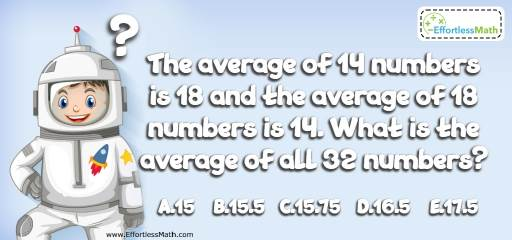# Algebra Puzzle – Challenge 45

Love to tease your brain with math and critical thinking challenges? If so, this is a great math puzzle for you.## Challenge:

The average of 14 numbers is 18 and the average of 18 numbers is 14. What is the average of all 32 numbers?

A- 15

B- 15.5

C- 15.75

D- 16.5

E- 17.5

### The Absolute Best Book to Challenge Your Smart Student!

The average of 14 numbers is 18. Therefore, the sum of 14 numbers is 252.
The average of 18 numbers is 14. Therefore, the sum of 18 numbers is 252. The sum of all 32 numbers is 504.
The average of 32 numbers is: 504 ÷ 32 = 15.75

The Absolute Best Books to Ace Algebra

### What people say about "Algebra Puzzle – Challenge 45 - Effortless Math: We Help Students Learn to LOVE Mathematics"?

No one replied yet.

X
45% OFF

Limited time only!

Save Over 45%

SAVE $40 It was$89.99 now it is \$49.99